﻿ Influence of Water | Slope Stability | GEO5 | Online Help
0Tree
Settings
Program:
Language:

## Influence of Water

Groundwater can be assigned to the slope plane section using one of the five options:

## 1) Groundwater table

The groundwater table is specified as a polygon. It can be arbitrarily curved, placed totally within the soil body or introduced partially above the ground surface.

Presence of water influences the value of pore pressure acting within the soil and reducing its shear bearing capacity. The pore pressure is considered as the hydrostatic pressure, i.e. unit weight of the water is multiplied by the reduced height of the water table: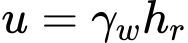where: γw - unit weight of water hr - reduced height of the water table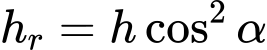where: h - vertical distance of point, where pore pressure is calculated and point on the water table α - inclination of the water table

Resultant force of pore pressure at a certain section of the block is used in the calculation: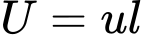where: u - pore pressure in the point l - length of section

Below the groundwater table, the analysis proceeds using the unit weight of saturated soil γsat and uplift pressure; above the groundwater table the analysis assumes the input unit weight of soil γ.

The shear forces along the slip surface are provided by: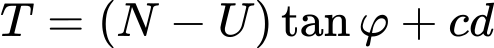where: T - shear force along the slip surface segment N - normal force along the slip surface segment U - pore pressure resultant along the slip surface segment φ - angle of the internal friction c - cohesion d - length of the slip surface segment

In case of a total stress (entered in the "Soil" dialog window) total parameters are used and pore pressure is considered zero.

## 2) Groundwater table including suction

The suction table can be introduced above the input groundwater table. A negative value of the pore pressure u is then assumed with the region separated by the two tables. Suction increases as a negative hydrostatic pressure from the groundwater table towards the suction table.

## 3) Rapid draw down

The original table can be introduced above the input groundwater table. The original water table simulates the state before rapid drawdown.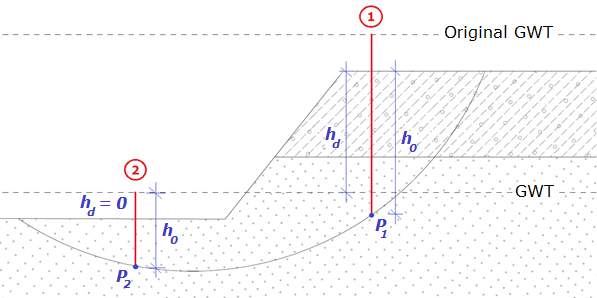Rapid draw down analysis

First of all, the initial pore pressure u0 is evaluated: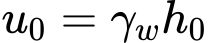where: h0 - height from the original table to the point of evaluation P γw - unit weight of water

Height h0 is generally the distance from the point of pore pressure evaluation (P) to original water table - this is valid for the case when the original water table is under the terrain surface. In case of original water table above terrain there is used the height h0 from point P to the level of terrain surface (profile 1 in the figure). Another case is the when original water table, as well as groundwater table are both above terrain - then height h0 is the distance from groundwater table to point P (profile 2 in the figure).

The second step is to calculate the change of pore pressure in the area between the original and groundwater table: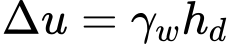where: hd - height from original to groundwater table γw - unit weight of water

As in previous calculation of pressure, there are three possibilities of how to get height hd. When both water tables are under the terrain, hd is the distance between the original and groundwater table. In case the original water table is above the terrain, then hd is measured from groundwater table to the level of terrain (profile 1 in the figure). Last case is when both water tables are above the terrain - then the height hd is zero (profile 2 in the figure).

Third step is the calculation of the final value of pore pressure u. Change of pore pressure Δu is multiplied by the coefficient of reduction of the initial pore pressure X, which is required for all soils (dialog window "Soils").  X coefficient of the soil in the area of point P is used (NOT the soil in the area between original and groundwater table). In the case of permeable soil X = 1, in other case X = 0. Final pore pressure is evaluated as: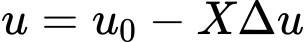where: u0 - initial pore pressure X - coefficient of reduction of the initial pore pressure Δu - change of pore pressure

## 4) Coefficient of pore pressure Ru

The coefficient of pore pressure Ru represents the ratio between the pore pressure and geostatic pressure in a soil body. In the area, where Ru is positive, entered unit weight of saturated soil γsat is considered; in other case unit weight of soil γ is used.

The values of Ru are introduced with the help of isolines connecting points with the same value of Ru. Linear interpolation is assumed to obtain intermediate values. Pore pressure is established as geostatic stress reduced by coefficient Ru:where: Ru - coefficient of pore pressure hi - height of ith soil layer γi - unit weight of ith soil layer

## 5) Pore pressure values

Groundwater can be introduced directly through the pore pressure values u within the plane section of a soil body.

In the area, where u is positive, entered unit weight of saturated soil γsat is considered; in other case unit weight of soil γ is used.

The pore pressure values are introduced with the help of isolines connecting points with the same value of pore pressure. Linear interpolation is assumed to obtain intermediate values. Pore pressure values are then derived from the values of pore pressure obtained in specific points within the slope plane section.

## 6) Waterflow analysis

The last option serves to analyze the pore pressures in the "Slope Stability - Water Flow" module. This option is available only for the users with the "Slope Stability - Water Flow" module purchased.